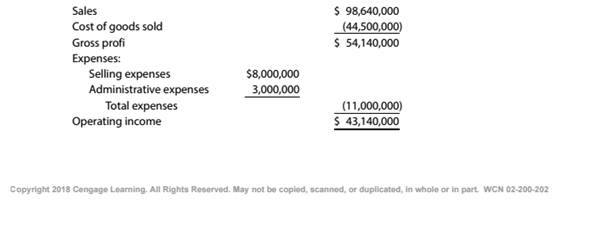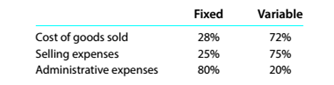Chapter 11, Problem 11.2.2P

Chapter
Section
Textbook Problem

Break-even sales under present and proposed conditions Kearney Company, operating at full capacity, sold 400,000 units at a price of \$246.60 per unit during 20Y5. Its income statement for 20Y5 is as follows:The division of costs between fixed and variable is as follows:Management is considering a plant expansion program that will permit an increase of \$8,631,000 (35.000 units at \$246.60) in yearly sales. The expansion will increase fixed costs by \$3,600,000 but will not affect the relationship between sales and variable costs.InstructionsDetermine for 20Y5 (a) the unit variable cost and (b) the unit contribution margin.

To determine

Concept Introduction:

Cost Volume Profit (CVP) Analysis:

The Cost Volume Profit analysis is the analysis of the relation between cost, volume, and profit of a product. It analyzes the cost and profits at the different level of production, in order to determine the breakeven point and required the level of sales to earn the desired profit.

Contribution margin means the margin that is left with the company after recovering variable cost out of revenue earned by selling smart phones. The formula:

Contribution margin = Sales - Variable cost.

Similarly contribution margin ratio = Contribution/sales

To Calculate:

The Unit variable cost and unit contribution margin

Explanation

The Unit variable cost and unit contribution margin are calculated as follows:

 Total Variable costs (A) \$ 38,640,000 Number of units Produced (B) 400,000

Still sussing out bartleby?

Check out a sample textbook solution.

See a sample solution

The Solution to Your Study Problems

Bartleby provides explanations to thousands of textbook problems written by our experts, many with advanced degrees!

Get Started

Why are actual overhead rates seldom used in practice?

Managerial Accounting: The Cornerstone of Business Decision-Making

INTEREST RATE PREMIUMS A 5-year Treasury bond has a 5.2% yield. A 10-year Treasury bond yields 6.4%, and a 10-y...

Fundamentals of Financial Management, Concise Edition (with Thomson ONE - Business School Edition, 1 term (6 months) Printed Access Card) (MindTap Course List)

Define net exports and net capital outflow. Explain how they are related.

Brief Principles of Macroeconomics (MindTap Course List)

IRR Refer to problem 11-1. What is the projects IRR?

Fundamentals of Financial Management (MindTap Course List)